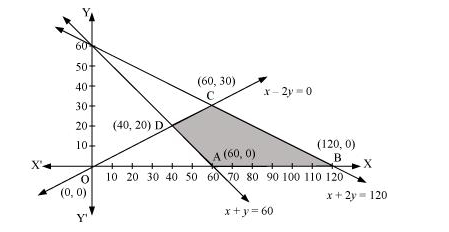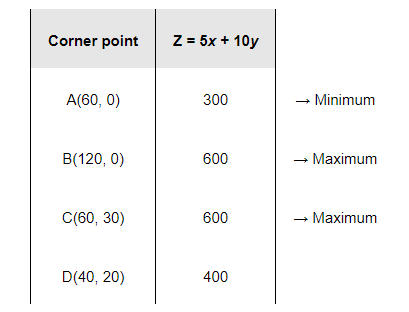# Minimise and Maximise

Question:

Minimise and Maximise $Z=5 x+10 y$

subject to $x+2 y \leq 120, x+y \geq 60, x-2 y \geq 0, x, y \geq 0$.

Solution:

The feasible region determined by the constraints, x + 2y ≤ 120, x + y ≥ 60, x − 2y ≥ 0, x ≥ 0, and y ≥ 0, is as follows.The corner points of the feasible region are A (60, 0), B (120, 0), C (60, 30), and D (40, 20).

The values of Z at these corner points are as follows.The minimum value of Z is 300 at (60, 0) and the maximum value of Z is 600 at all the points on the line segment joining (120, 0) and (60, 30).